# A ball of mass M, moving with speed V, strikes the end of a motionless, uniform...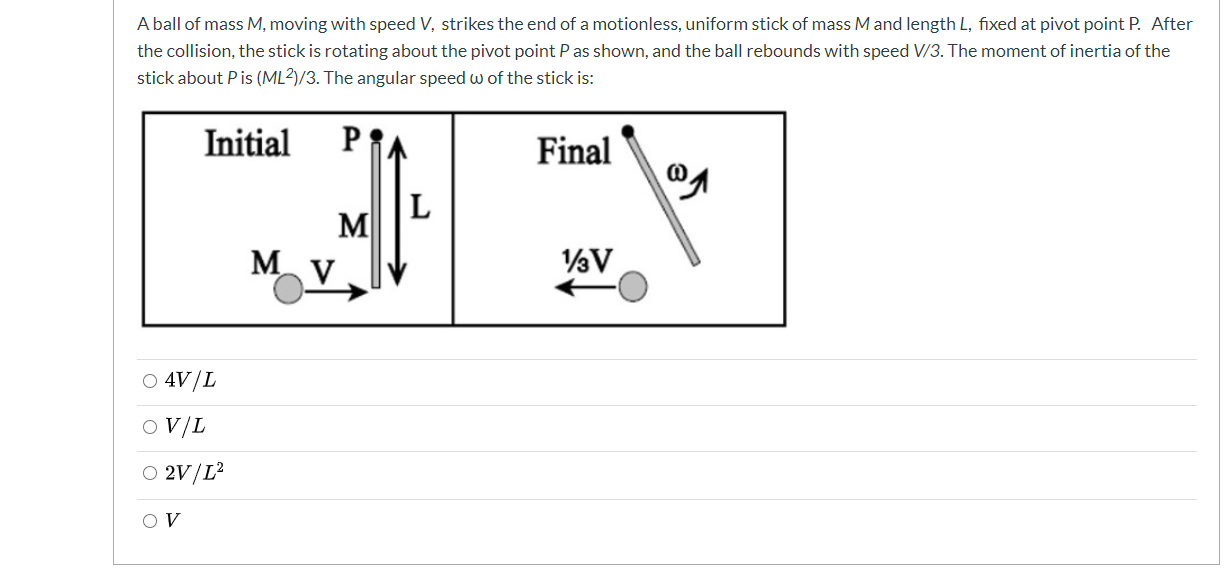A ball of mass M, moving with speed V, strikes the end of a motionless, uniform stick of mass Mand length L, fixed at pivot point P. After the collision, the stick is rotating about the pivot point P as shown, and the ball rebounds with speed V/3. The moment of inertia of the stick about Pis (ML2/3. The angular speed w of the stick is: Initial P Final L M MV VV O 4V/L OV/L O2V/L OV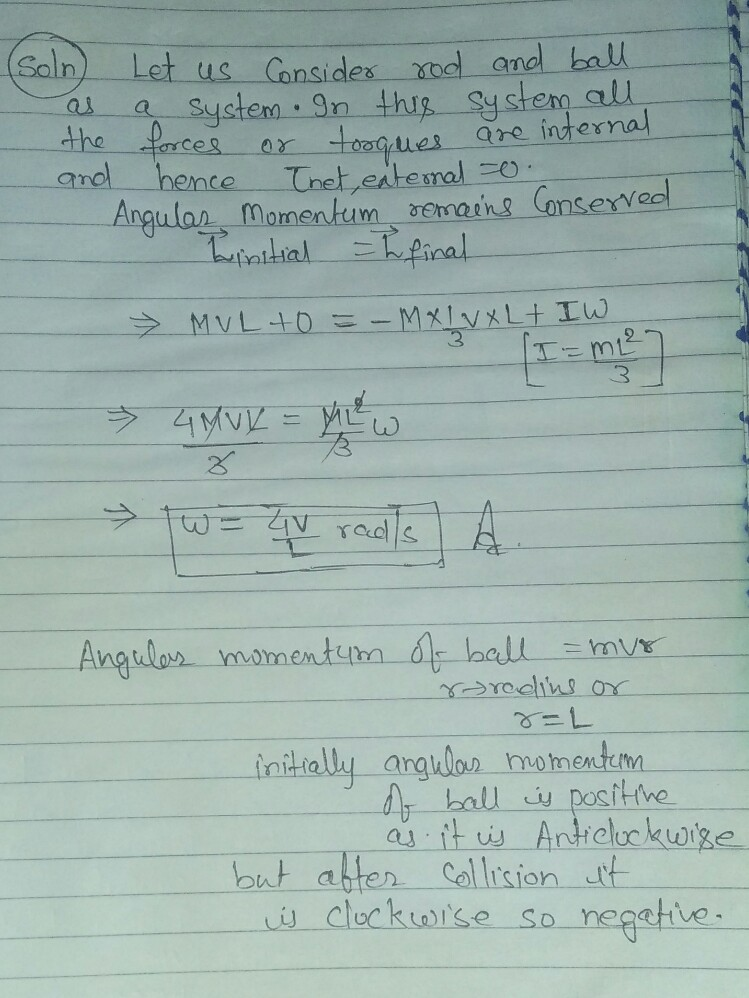##### Add Answer of: A ball of mass M, moving with speed V, strikes the end of a motionless, uniform...
Similar Homework Help Questions
• ### Q20 (15 points): A bullet of mass m X102 kg is moving with a speed of...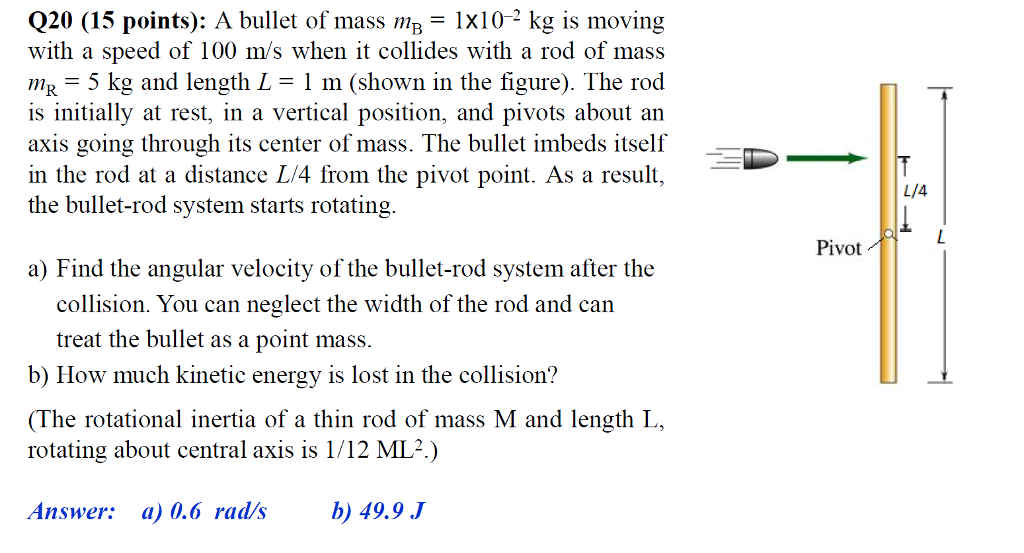Q20 (15 points): A bullet of mass m X102 kg is moving with a speed of 100 m/s when it collides with a rod of mass mR 5 kg and length L m (shown in the figure). The rod is initially at rest, in a vertical position, and pivots about an axis going through its center of mass. The bullet imbeds itself in the rod at a distance L/4 from the pivot point. As a result, the bullet-rod system starts...

• ### A ball of mass m made of playdough moves with speed v. Another ball, of the...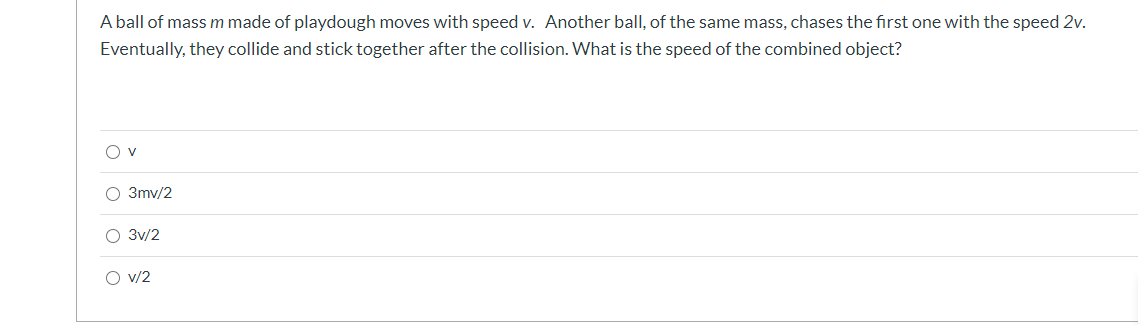A ball of mass m made of playdough moves with speed v. Another ball, of the same mass, chases the first one with the speed 2v. Eventually, they collide and stick together after the collision. What is the speed of the combined object? Ov O 3mv/2 O 3v/2 O v/2

• ### (17%) Problem 2: A uniform wooden meter stick has a mass of m= 799 g. A...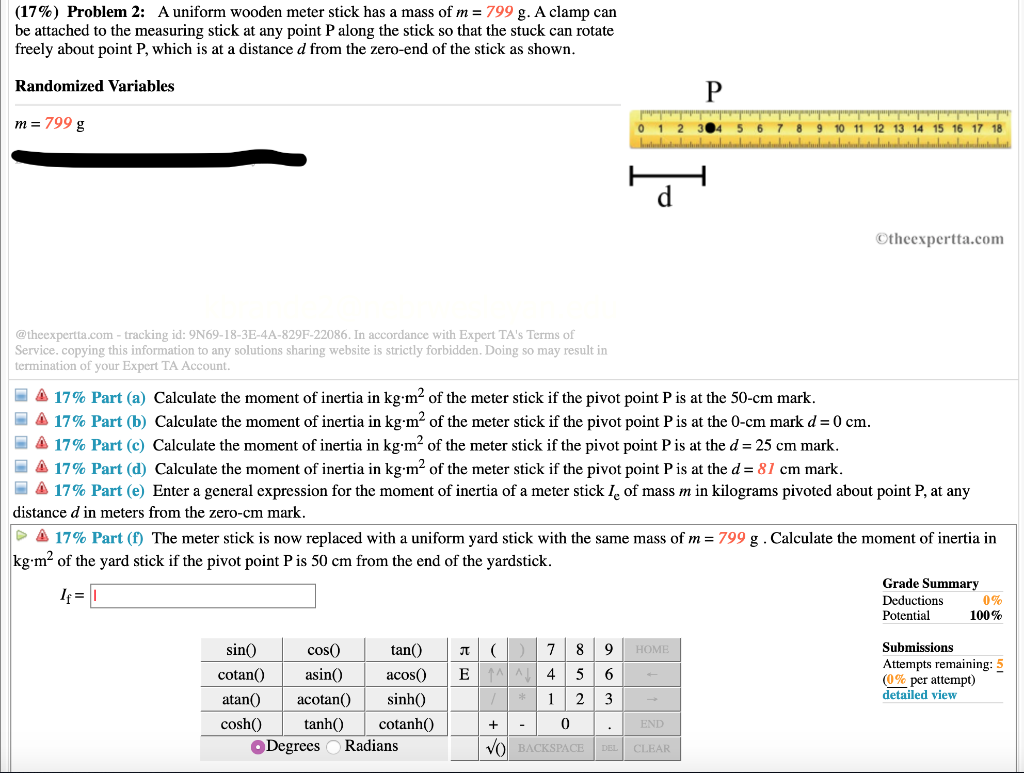(17%) Problem 2: A uniform wooden meter stick has a mass of m= 799 g. A clamp can be attached to the measuring stick at any point P along the stick so that the stuck can rotate freely about point P, which is at a distance d from the zero-end of the stick as shown. Randomized Variables m = 799 g 304 5 6 7 8 9 10 11 12 13 14 15 16 17 18 LLLLLLLL LLLLLLLL Otheexpertta.com @theexpertta.com...

• ### 5. A ball of mass m strikes a massive wall at speed v, at an angle...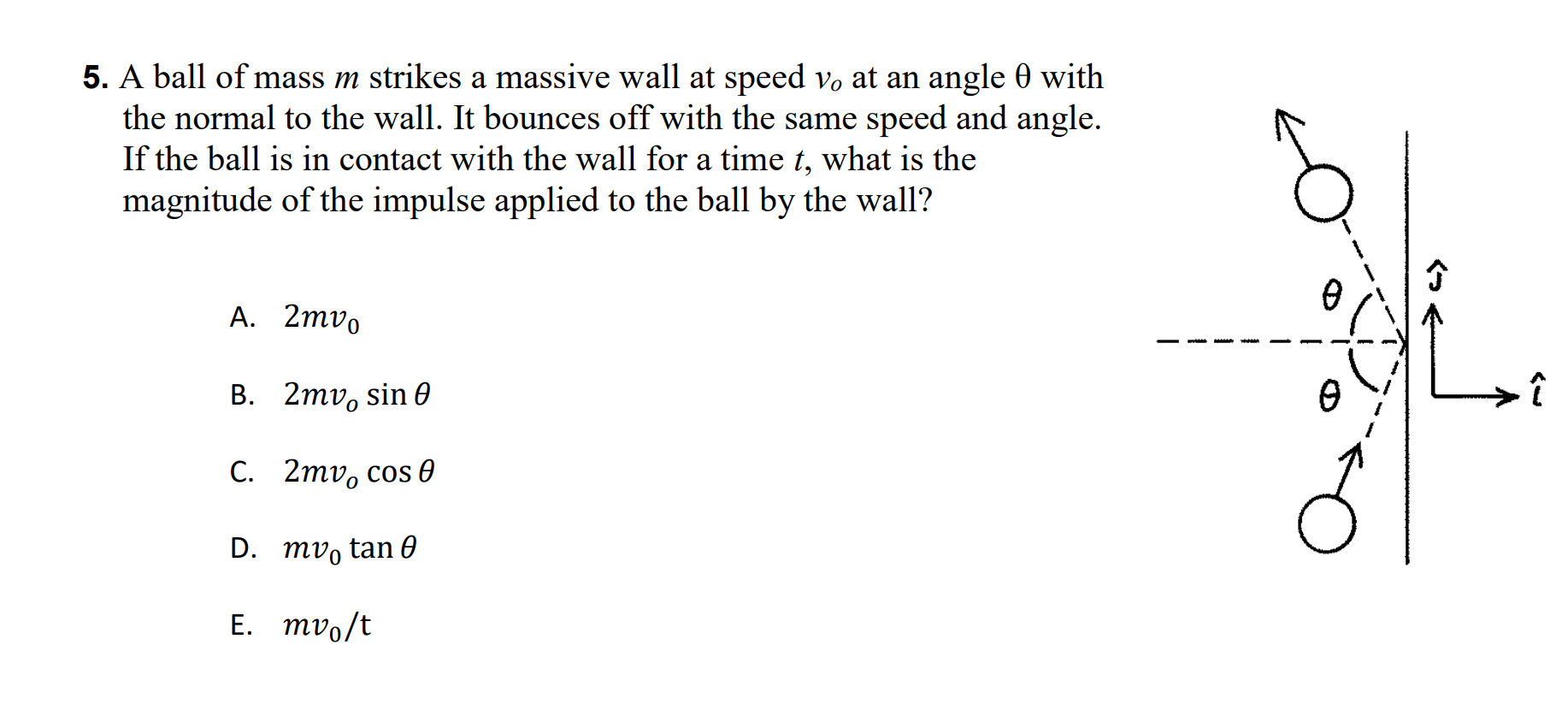5. A ball of mass m strikes a massive wall at speed v, at an angle 0 with the normal to the wall. It bounces off with the same speed and angle. If the ball is in contact with the wall for a time t, what is the magnitude of the impulse applied to the ball by the wall? A. 2mv. B. 2mv, sino OL→î C. 2mv, cos e D. mv, tan o E. mv./t

• ### A tennis ball of mass m = 0.060 kg and speed v = 22 m/s strikes a wall at a 45^circ angle and...

A tennis ball of mass m = 0.060 kg and speed v = 22 m/s strikes a wall at a 45^circ angle and rebounds with the same speed at 45 degrees. What is the magnitude of theimpulse given to the ball?

• ### A tennis ball of mass m = 0.065 kg and speed v = 65 m/s strikes a wall at a 45° angle and...

A tennis ball of mass m = 0.065 kg and speed v = 65 m/s strikes a wall at a 45° angle and rebounds with the same speed at 45° (Fig. 7-29). What is the impulse giventhe wall?

• ### A bullet of mass m = 40 g, moving horizontally with speed v, strikes a clay...

A bullet of mass m = 40 g, moving horizontally with speed v, strikes a clay block of mass M=1.33 kg that is hanging on a light inextensible string of length L=0.767. The bullet becomes embedded in the block, which was originally at rest. What is the smallest value of vv which would cause the block-on-a-string to swing around and execute a complete vertical circle?

• ### A uniform rod of mass m-6 kg has length L = 60 cm . Instead of...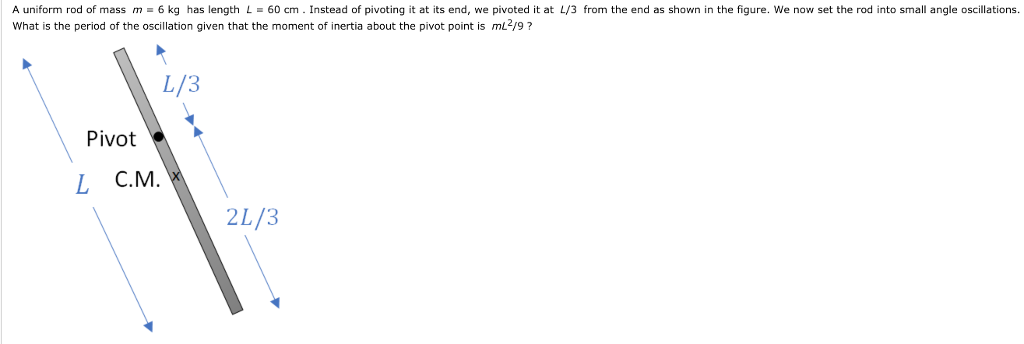A uniform rod of mass m-6 kg has length L = 60 cm . Instead of pivoting it at its end, we pivoted it at L/3 from the end as shown in the figure, we now set the rod into small angle oscillations. What is the period of the oscillation given that the moment of inertia about the pivot point is ml2/9? L/3 Pivot L С.М. 2L/3

• ### A uniform rod of mass m- 9 kg has length L-70 cm. Instead of pivoting it...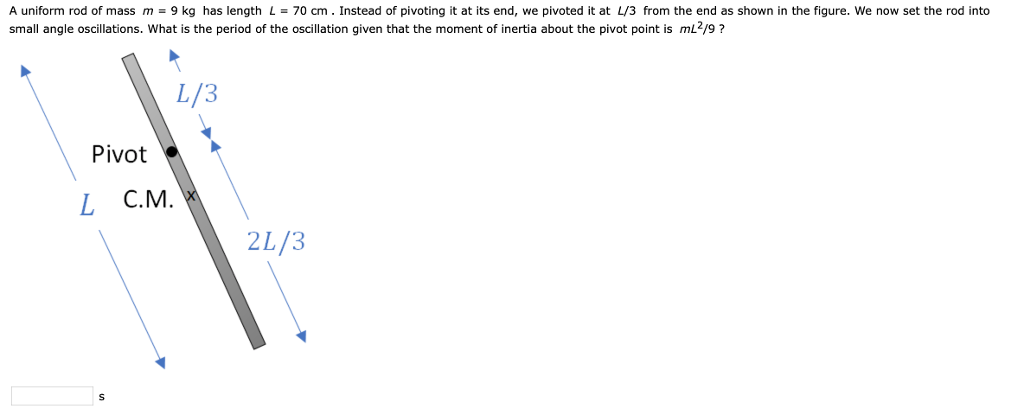A uniform rod of mass m- 9 kg has length L-70 cm. Instead of pivoting it at its end, we pivoted it at L/3 from the end as shown in the figure. We now set the rod into small angle oscillations. What is the period of the oscillation given that the moment of inertia about the pivot point is mL2/9? L/3 Pivot L с.м. 2L/3

• ### 8. A ball of mass m strikes a wall perpendicularly with a speed v, and it...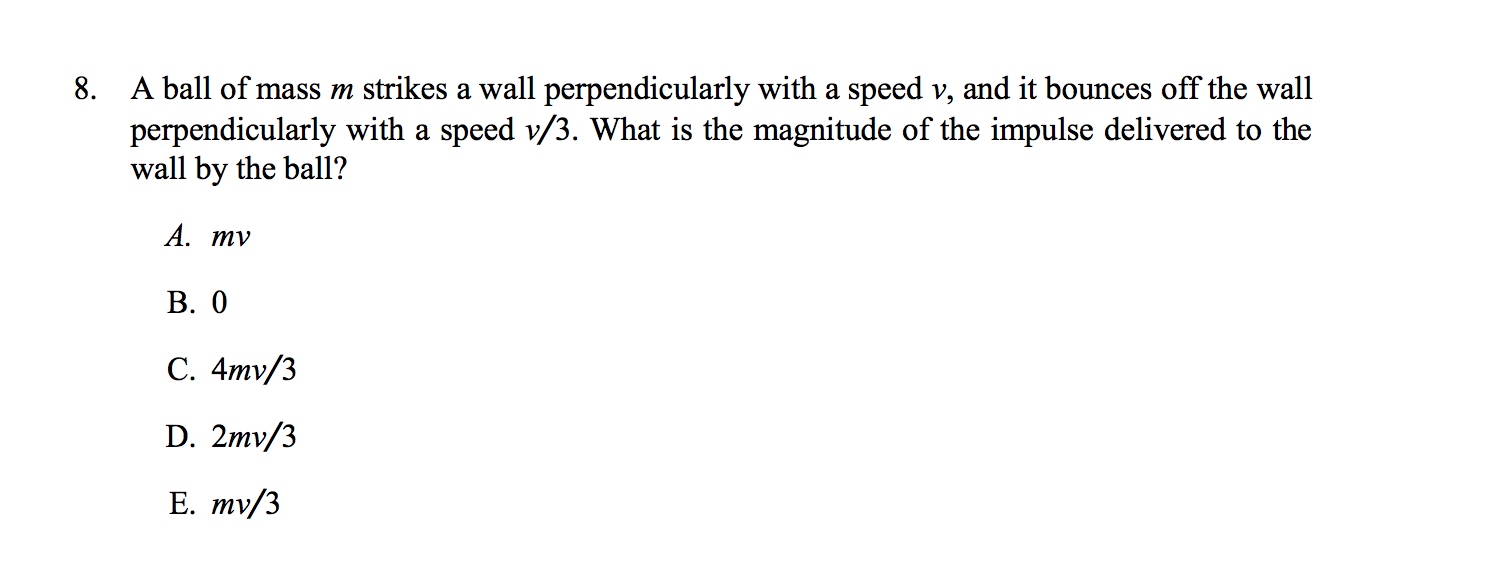8. A ball of mass m strikes a wall perpendicularly with a speed v, and it bounces off the wall perpendicularly with a speed v/3. What is the magnitude of the impulse delivered to the wall by the ball? А. ту В. О C. 4mv/3 D. 2mv/3 Е. тy/3

Free Homework App